Checkout JEE MAINS 2022 Question Paper Analysis : Checkout JEE MAINS 2022 Question Paper Analysis :

# Algebraic Integrals

Finding the solution of algebraic integrals is a lengthy and complex process. Hence, a standard method is to be followed to attain the solution in an easy way. In this section, we will discuss different forms of algebraic integrals and tricks given will help aspirants to solve all the problems at their own pace.

The types of integrals are as follows:

• The integral can be called a definite integral if the function f(x) is between the limits ‘a’ and ‘b’, where d/dx (F(x)) = f(x).
 It is represented as follows: $$\begin{array}{l}\int_{a}^{b}f(x)\end{array}$$ dx = F(b) – F(a)
• Indefinite integrals refer to the evaluation of the indefinite area. The diagram below explains the difference between definite and indefinite integral.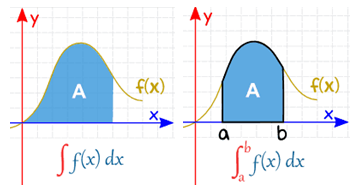## Algebraic Integrals Forms

The following forms of integrals can be categorised into algebraic integrals.

1]

$$\begin{array}{l}\int \frac{1}{ax^2+bx+c}dx\end{array}$$
or reducible to
$$\begin{array}{l}\int \frac{1}{ax^2+bx+c}dx\end{array}$$

Tricks to Solve: In these integrals try expressing the term ax2 + bx + c as sum or difference of the two squares. The following steps can be followed:

• By dividing or multiplying throughout, the coefficient of x2 can be made unity.
• To express it in the form
$$\begin{array}{l}[x+\frac{b}{2a}]^2\end{array}$$
+
$$\begin{array}{l}\frac{4ac-b^2}{4a^2}\end{array}$$
, add and subtract the square of half of the coefficient of x.
• This reduces to into one of the forms and can be easily integrated.
$$\begin{array}{l}\frac{1}{x^2-a^2}\ or\ \frac{1}{x^2+a^2}\ or\ \frac{1}{a^2-x^2}\end{array}$$

2]

$$\begin{array}{l}\int \frac{1}{\sqrt{ax^2+bx+c}}dx\end{array}$$
or reducible to
$$\begin{array}{l}\int \frac{1}{\sqrt{ax^2+bx+c}}dx\end{array}$$

Tricks to Solve: For integrating these functions the following steps can be followed.

• Make the coefficient of x2 as unity.
• To express the term within the square root in the form
$$\begin{array}{l}[x+\frac{b}{2a}]^2\end{array}$$
+
$$\begin{array}{l}\frac{4ac-b^2}{4a^2}\end{array}$$
, Add and subtract the square of half of the coefficient of x.
• This reduces to into one of the forms and can be easily integrated.
$$\begin{array}{l}\frac{1}{x^2-a^2}\ or\ \frac{1}{x^2+a^2}\ or\ \frac{1}{a^2-x^2}\end{array}$$

3]

$$\begin{array}{l}\int \frac{px+q}{ax^2+bx+c}\end{array}$$

Tricks to Solve:

• In such cases, the numerator can be expressed as the sum of constant times differentiation of denominator and a constant that is, px + q is written as A (2ax + b) + C, where 2ax + b= d/dx (ax2+bx+c). A and C are arbitrary constants.
• By equating on the coefficients of like powers on both sides, the values of the constants can be easily obtained.
• Replace the numerator (px + q) by A (2ax + b) + C in the given integral which will give,
$$\begin{array}{l}\int\frac{px+q}{ax^2+bx+c}=A\int\frac{2ax+b}{ax^2+bx+c}dx+C\int\frac{1}{ax^2+bx+c}dx\end{array}$$
• The right hand side value thus obtained in the previous step can be integrated and then constant values can be substituted.

4]

$$\begin{array}{l}\int\frac{px+q}{\sqrt{ax^2+bx+c}}\end{array}$$

Tricks to Solve:

In this case, we proceed as follows:

• The numerator is expressed as the sum of constant times differentiation of denominator and a constant, that is, px + q is written as A (2ax + b) + C, where 2ax + b=d/dx (ax2+bx+c). A and C are arbitrary constants.
• The values of constants are computed by equating the coefficients of like powers on both sides.
• Replace the numerator (px + q) by A (2ax + b) + C in the given integral which will give,
$$\begin{array}{l}\int\frac{px+q}{\sqrt{ax^2+bx+c}}dx=A\int\frac{2ax+b}{\sqrt{ax^2+bx+c}}dx+C\int \frac{1}{\sqrt{ax^2+bx+c}}dx\end{array}$$
• The right hand side value thus obtained in the previous step can be integrated and then constant values can be substituted.

5]

$$\begin{array}{l}\int \sqrt{ax^2+bx+c}dx\end{array}$$

Tricks to Solve:

In such type of integrals, the following steps are followed:

• Make the coefficient of x2 as unity.
• To obtain the form
$$\begin{array}{l}[x+\frac{b}{2a}]^2\end{array}$$
+
$$\begin{array}{l}\frac{4ac-b^2}{4a^2}\end{array}$$
, Add and subtract the square of half of the coefficient of x.
• Henceforth the integral reduces to,
$$\begin{array}{l}\int \sqrt{a^2+x^2}dx \end{array}$$
, or
$$\begin{array}{l}\int \sqrt{a^2-x^2}dx\end{array}$$

$$\begin{array}{l}\int \sqrt{x^2-a^2}dx\end{array}$$
• These integrals can be computed easily by using direct formulae.

Definite and indefinite integration

Integration of functions

Applications of integration

## Algebraic Integrals Examples

Below are some solved examples on algebraic integrals for IIT JEE aspirants.

Example 1:

$$\begin{array}{l}\int \frac{x+1}{\sqrt{2x^2+x-3}}dx\end{array}$$

Solution:

By completing the square,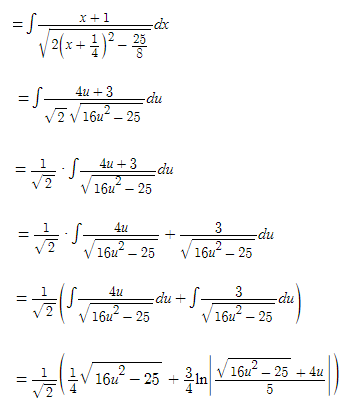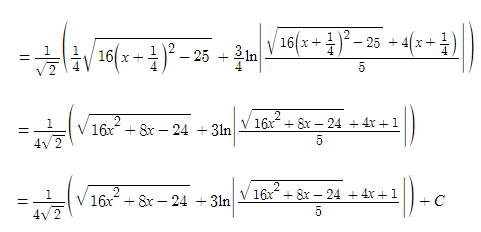Example 2:

$$\begin{array}{l}\int \frac{1}{x^2+6x+5}dx\end{array}$$

Solution: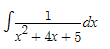By completing the square,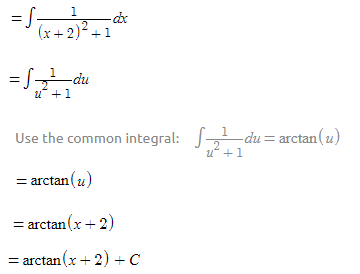Example 3:

$$\begin{array}{l}\int \frac{1}{\sqrt{6x^2-5x+1}}dx\end{array}$$

Solution: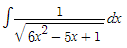By completing the square,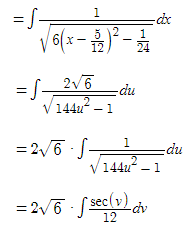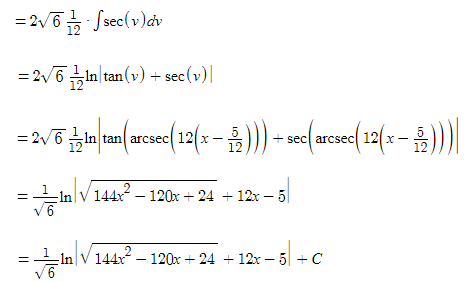Example 4:

$$\begin{array}{l} I=\int _0^{10}\left|sinx\right|\:dx\\\end{array}$$

Solution:

$$\begin{array}{l}=\int _0^{\pi }\sin \left(x\right)dx+\int _{\pi }^{2\pi }-\sin \left(x\right)dx+\int _{2\pi }^{3\pi }\sin \left(x\right)dx+\int _{3\pi }^{10}-\sin \left(x\right)dx\\\int _0^{\pi }\sin \left(x\right)dx=\left[-\cos \left(x\right)\right]^{\pi }_0=2\\ \int _{\pi }^{2\pi }-\sin \left(x\right)dx=-\int _{\pi }^{2\pi }\sin \left(x\right)dx=-\left[-\cos \left(x\right)\right]^{2\pi }_{\pi }=-\left(-2\right)\\\int _{2\pi }^{3\pi }\sin \left(x\right)dx=\left[-\cos \left(x\right)\right]^{3\pi }_{2\pi }=2\\\int _{3\pi }^{10}-\sin \left(x\right)dx=-\left[-\cos \left(x\right)\right]^{10}_{3\pi }=-\left(-\cos \left(10\right)-1\right)\\=2+2+2+\cos \left(10\right)+1\\=\cos \left(10\right)+7\end{array}$$

Example 5: If

$$\begin{array}{l}I_{n}=\int_{0}^{\pi/4}tan^{n}xdx\end{array}$$
, then
$$\begin{array}{l}\lim_{n\rightarrow \infty}n[I_{n}+I_{n+2}]=\end{array}$$

Solution:

$$\begin{array}{l}I_{n}+I_{n+2}=\int_{0}^{\pi/4}tan^{n}x[1+tan^{2}xdx]\\ \int_{0}^{\pi/4}tan^{n}xsec^{2}xdx=\frac{tan^{n+1}x}{n+1} \text \ from \ 0 \ to \ \frac{\pi}{4}\\ =\frac{1-0}{n+1}=\frac{1}{n+1}\\ I_{n}+I_{n+2}=\frac{1}{n+1}\\\Rightarrow \lim_{x\rightarrow \infty }n[I_{n}+I_{n+2}]\\ \Rightarrow \lim_{x\rightarrow \infty }n*\frac{1}{n+1}=\frac{n}{n+1}=\frac{n}{n*[1+\frac{1}{n}]}=1\end{array}$$

Example 6:

$$\begin{array}{l}\int _0^1\sqrt{1+7x}dx\end{array}$$

Solution:

$$\begin{array}{l}\mathrm{Apply\:u-substitution:}\:u=1+7x\\=\int _1^8\frac{\sqrt{u}}{7}du\\=\frac{1}{7}\cdot \int _1^8\sqrt{u}du\\=\frac{1}{7}\cdot \int _1^8u^{\frac{1}{3}}du\\=\frac{1}{7}\left[\frac{u^{\frac{1}{3}+1}}{\frac{1}{3}+1}\right]^8_1\\=\frac{1\cdot \:\left[\frac{u^{\frac{1}{3}+1}}{\frac{1}{3}+1}\right]^8_1}{7}\\\frac{u^{\frac{1}{3}+1}}{\frac{1}{3}+1}=\frac{3u^{\frac{4}{3}}}{4}\\=\frac{\left[\frac{u^{\frac{4}{3}}\cdot \:3}{4}\right]^8_1}{7}\\=\frac{1}{7}\left[\frac{u^{\frac{4}{3}}\cdot \:3}{4}\right]^8_1\\=\frac{1}{7}\cdot \frac{45}{4}\\=\frac{45}{28}\end{array}$$

Example 7:

$$\begin{array}{l}\int \frac{e^x}{e^x+e^{-x}}dx\end{array}$$

Solution:

$$\begin{array}{l}\int \frac{e^x}{e^x+e^{-x}}dx\\ \mathrm{Apply\:u-substitution:}\:u=e^x\\ =\int \frac{u}{u^2+1}du\\ \mathrm{Apply\:u-substitution:}\:v=u^2+1\\=\int \frac{1}{2v}dv\\\frac{1}{2}\ln \left|v\right|\\=\frac{1}{2}\ln \left|\left(e^x\right)^2+1\right|\\=\frac{1}{2}\ln \left|e^{2x}+1\right|\\=\frac{1}{2}\ln \left|e^{2x}+1\right|+C\end{array}$$

## Integrations Important JEE Main Questions### Definite Integration JEE Questions### Indefinite Integration JEE Questions### Name some methods of integration.

Integration by parts, integration by substitution, integration using trigonometric identities, integration by partial fraction are some methods of integration.

### What is the value of ∫aa f(x) dx?

The value of ∫aa f(x) dx = 0.

### If f(x) is an odd function, what is ∫–aa f(x) dx?

If f(x) is an odd function, ∫aa f(x) dx = 0.

### If f(x) is an even function, what is ∫–aa f(x) dx?

If f(x) is an even function, ∫aa f(x) dx = 2∫0a f(x) dx.